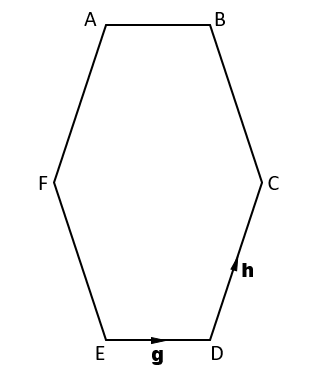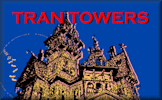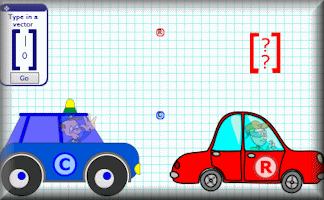Vectors

An online exercise on addition and subtraction of vectors and diagrammatic representations of vectors.

Level 1Level 2Level 3Exam-Style Description Help More VectorsThe diagram shows an irregular hexagon ABCDEF which has vertical and horizontal lines of symmetry. Opposite sides of the hexagon are parallel. $$\overrightarrow{ED} = \pmb{g}$$ $$\overrightarrow{DC} = \pmb{h}$$ The diagram is not to scale.

1. Let's begin with a nice easy, straight-forward question. Choose the option below which shows the vector representation of $$\overrightarrow{EC}$$ in terms of $$\pmb{g}$$ and $$\pmb{h}$$.

 A .     $$\pmb{g+h}$$ B .     $$\pmb{g-h}$$ C .     $$\pmb{h-g}$$ D .     $$\pmb{2g}$$ E .     $$\pmb{g+\frac12 h}$$2. Find $$\overrightarrow{BF}$$ in terms of $$\pmb{g}$$ and $$\pmb{h}$$.

 A .     $$\pmb{g+h}$$ B .     $$\pmb{g-h}$$ C .     $$\pmb{-g-h}$$ D .     $$\pmb{h-g}$$ E .     $$\pmb{h}$$3. The ratio of FC to ED is 5:2. Find $$\overrightarrow{FC}$$ in terms of $$\pmb{g}$$.

 A .     $$\pmb{5g}$$ B .     $$\pmb{2g}$$ C .     $$\pmb{7g}$$ D .     $$\pmb{\frac{2}{5}g}$$ E .     $$\pmb{\frac{5}{2}g}$$4. Find $$\overrightarrow{EF}$$ in terms of $$\pmb{g}$$ and $$\pmb{h}$$.

 A .     $$\pmb{2h-3g}$$ B .     $$\pmb{h-\frac{3}{2}g}$$ C .     $$\pmb{3h-2g}$$ D .     $$\pmb{h-\frac{2}{3}g}$$ E .     $$\pmb{7h-5g}$$5. The point M is the midpoint of DC. Find $$\overrightarrow{EM}$$ in terms of $$\pmb{g}$$ and $$\pmb{h}$$.

 A .     $$\pmb{g + \frac12 h}$$ B .     $$\pmb{h + \frac12 g}$$ C .     $$\pmb{2g + h}$$ D .     $$\pmb{h + 2g}$$ E .     $$\pmb{\frac12 g + \frac12 h}$$6. The point N lies on ED such that the ratio of EN to ND is 1:2. Find $$\overrightarrow{CN}$$ in terms of $$\pmb{g}$$ and $$\pmb{h}$$.

 A .     $$\pmb{-h -g + \frac12 g}$$ B .     $$\pmb{-3g - 2h}$$ C .     $$\pmb{2g - 2h}$$ D .     $$\pmb{-h-\frac23 g}$$ E .     $$\pmb{-h-\frac12 g}$$7. The point P lies on FA such that the ratio FP to PA is 4:3. Find $$\overrightarrow{CP}$$ in terms of $$\pmb{g}$$ and $$\pmb{h}$$.

 A .     $$\pmb{\frac{3}{4}g + \frac{2}{5}h}$$ B .     $$\pmb{\frac{4}{3}g - \frac{5}{2}h}$$ C .     $$\pmb{\frac{4}{7}g + \frac{5}{2}h}$$ D .     $$\pmb{\frac{4}{7}g - \frac{5}{2}h}$$ E .     $$\pmb{\frac{4}{7}h - \frac{5}{2}g}$$8. Find $$\overrightarrow{NP}$$ in terms of $$\pmb{g}$$ and $$\pmb{h}$$.

 A .     $$\pmb{11(\frac{h}{7} - \frac{g}{6})}$$ B .     $$\pmb{11(\frac{g}{7} - \frac{h}{6})}$$ C .     $$\pmb{11(\frac{g}{7} + \frac{h}{6})}$$ D .     $$\pmb{11(\frac{h}{7} + \frac{g}{6})}$$ E .     $$\pmb{\frac{11}{7}h + \frac{11}{6}g}$$9. Find $$\overrightarrow{PM}$$ in terms of $$\pmb{g}$$ and $$\pmb{h}$$.

 A .     $$\pmb{5(\frac{g}{2}+\frac{3h}{14})}$$ B .     $$\pmb{5(\frac{g}{2}-\frac{3h}{14})}$$ C .     $$\pmb{\frac{5g}{2}+\frac{15h}{14}}$$ D .     $$\pmb{5(\frac{h}{2}+\frac{3g}{14})}$$ E .     $$\pmb{\frac{5h}{2}+\frac{15g}{14}}$$10. The line AB is extended to X such that the ration AB to AX is 2:7. Find $$\overrightarrow{EX}$$ in terms of $$\pmb{g}$$ and $$\pmb{h}$$.

 A .     $$\pmb{\frac{2}{7}h + \frac{2}{5}g}$$ B .     $$\pmb{7h-5g}$$ C .     $$\pmb{-h-\frac12 g}$$ D .     $$\pmb{\frac{5h}{2}+\frac{15g}{14}}$$ E .     $$\pmb{2(g+h)}$$This is Vectors level 3. You can also try:
Level 1 Level 2

Instructions

Try your best to answer the questions above. Choose one of the five possible answers. When you have finished click the "check" button. If you have any questions wrong, do your best to do corrections but if there is anything you don't understand, please ask your teacher for help.

When you have got all of the questions correct you may want to print out this page and paste it into your exercise book. If you keep your work in an ePortfolio you could take a screen shot of your answers and paste that into your Maths file. You can also claim a 'Transum Trophy' by completing this quiz.

Transum.org

This web site contains hundreds of free mathematical activities for teachers and students. Click here to go to the main page which links to all of the resources available.More Activities:

Comment recorded on the 1 May 'Starter of the Day' page by Phil Anthony, Head of Maths, Stourport High School:

"What a brilliant website. We have just started to use the 'starter-of-the-day' in our yr9 lessons to try them out before we change from a high school to a secondary school in September. This is one of the best resources on-line we have found. The kids and staff love it. Well done an thank you very much for making my maths lessons more interesting and fun."

Comment recorded on the 14 September 'Starter of the Day' page by Trish Bailey, Kingstone School:

"This is a great memory aid which could be used for formulae or key facts etc - in any subject area. The PICTURE is such an aid to remembering where each number or group of numbers is - my pupils love it!
Thanks"

Teacher! Are you delivering Maths lessons online?

Parents! Has home-schooling been thrust upon you at short notice?

Stay safe and wash yout hands!

Tran TowersA mathematical adventure game in the enigmatic home of Transum. Create your own map as you go deeper and deeper into this maze of rooms looking for the clues to find the treasure room.

There are answers to this exercise but they are only available to teachers who have subscribed to Transum and are currently signed in on this computer.

A Transum subscription unlocks the answers to most of the student online exercises, quizzes and puzzles. It also provides the teacher with access to quality external links on each of the Transum topic pages so that teachers can easily find the excellent resources we have found and add to the collection themselves.

Class lists, lesson plans and assessment data can also be stored in the Class Admin application and the teacher also has access to the Transum Trophies earned by class members.

Go Maths

Learning and understanding Mathematics, at every level, requires learner engagement. Mathematics is not a spectator sport. Sometimes traditional teaching fails to actively involve students. One way to address the problem is through the use of interactive activities and this web site provides many of those. Click here for more activities designed for students in upper Secondary/High school.

Teachers

If you found this activity useful don't forget to record it in your scheme of work or learning management system. The short URL, ready to be copied and pasted, is as follows:

Alternatively, if you use Google Classroom, all you have to do is click on the green icon below.

Do you have any comments? It is always useful to receive feedback and helps make this free resource even more useful for those learning Mathematics anywhere in the world. Click here to enter your comments.

Description of Levels

CloseLevel 1 - Addition and subtraction of vectors and multiplication of vectors by a scalar.

Level 2 - Diagrammatic representations and the modulus of vectors.

Level 3 - Diagrammatic representations and ratios.

Exam Style Questions - A collection of problems in the style of GCSE or IB/A-level exam paper questions (worked solutions are available for Transum subscribers).

More Vectors A link to the curriculum page for vectors.

Answers to this exercise are available lower down this page when you are logged in to your Transum account. If you don’t yet have a Transum subscription one can be very quickly set up if you are a teacher, tutor or parent.

And now for something completely different. Help the cops catch the robbers by finding the vectors that will end the chase.

Transum.org/go/?to=VectorcopsExample

The video above is from Maths Genie.

Don't wait until you have finished the exercise before you click on the 'Check' button. Click it often as you work through the questions to see if you are answering them correctly. You can double-click the 'Check' button to make it float at the bottom of your screen.

Answers to this exercise are available lower down this page when you are logged in to your Transum account. If you don’t yet have a Transum subscription one can be very quickly set up if you are a teacher, tutor or parent.

Close